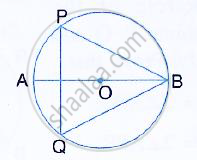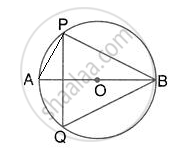Share

The Given Figure Shows a Circle with Centre O and ∠Abp = 42°Calculate the Measure Of: (I) ∠Pqb (Ii) ∠Qpb + ∠Pbq - ICSE Class 10 - Mathematics

ConceptArc and Chord Properties - Angles in the Same Segment of a Circle Are Equal (Without Proof)

Question

The given figure shows a circle with centre O and ∠ABP = 42°Calculate the measure of:
(i) ∠PQB
(ii) ∠QPB + ∠PBQ

SolutionJoin AP.
(i) ∠APB = 90°
(Angle in a semicircle)
∴ ∠BAP = 90° - ∠ABP = 90° - 42° = 48°
Now, ∠PQB = ∠BAP = 48°
(Angle in the same segment)

(ii) By angle sum property of ∆BPQ,
∠QPB + ∠PBQ = 180° - ∠PQB = 180° - 48° = 132°

Is there an error in this question or solution?
Solution The Given Figure Shows a Circle with Centre O and ∠Abp = 42°Calculate the Measure Of: (I) ∠Pqb (Ii) ∠Qpb + ∠Pbq Concept: Arc and Chord Properties - Angles in the Same Segment of a Circle Are Equal (Without Proof).
S